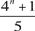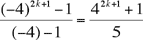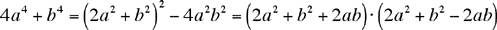Math Central - mathcentral.uregina.caProblem of the MonthCurrentproblem Recent problemswith solutions
 Older problems from 2005/2006 06/07 07/08 08/09 09/10 10/11 11/12

Solution to September 2005 Problem

The Problem:
Our first problem of the new season deals with integers.  As a warm-up exercise after the long summer break you might try to show that for every odd number n, 4n + 1 is a multiple of 5. For the September problem you must

Prove that if the number n is odd and greater than 3 thencannot be a prime number.

We received solutions (which were mostly correct, except for some problems with details, no doubt due to the summer break) from

 Said Amghibech (Quebec) Pierre Bornsztein (France) John Campbell (Edmonton) Xavier Hecquet (France) Wolfgang Kais (Germany) Patrick LoPresti (USA) Juan Mir Pieras (Spain)

Proof that 4n + 1 is divisible by 5 when n is odd.

One easy way to prove this is to show that the number is congruent to zero modulo 5:

When n is odd,

4nn(–1)n = (–1)2k+1 = –1 (mod 5), so that 4n +10 (mod 5),

as desired.

Alternatively, you can note that odd powers of 4 always end in 4 (41 = 4, 43 = 64, 45 = 1024, etc.).  It follows that 42k+1 + 1 ends in 5 and is therefore divisible by 5.

For yet another approach, Mir and Hecquet both used the geometric sum formula for r = –4:

S = 1 + (–4) + (–4)2 + ... + (–4)2k =; therefore 42k+1 + 1 = 5S so that 5 must divide evenly into  42k+1 + 1.

Proof that for n odd and greater than 3, (4n + 1)/5 cannot be a prime number.

All solutions were based on factoring 4a4 + b4.  We discussed factoring fourth-degree polynomials in general (and this polynomial in particular) as part of the solution to MP41 in April 2004.  Indeed,To apply this factorization to 4n + 1, we note that we can write n = 2k+1, so that

4n + 1 = 42k+1 + 1 = 4·42k + 1 = 4·24k + 1

= (2·22k +1 + 2·2k)(2·22k +1 – 2·2k).

But (2·22k +1 + 2·2k) > (2·22k +1 – 2·2k), and the smaller factor is bigger than 5, which can be seen as follows:

2·22k +1 – 2·2k = 2k+1(2k– 1) + 1 > 22(21– 1) + 1 = 5.

Thus, when n = 2k + 1 > 3, we have k > 1; this means that 4n + 1 factors into a product of two numbers that are both strictly greater than 5, so that dividing either of these numbers by 5 leaves it greater than 1.   By our preliminary exercise we saw that 4n + 1 is divisible by 5.  We conclude that (4n + 1)/5 itself has two integer factors that are both greater than 1.  In other words, it cannot be prime.

 Math Central is supported by the University of Regina and the Pacific Institute for the Mathematical Sciences.about math central :: site map :: links :: notre site français